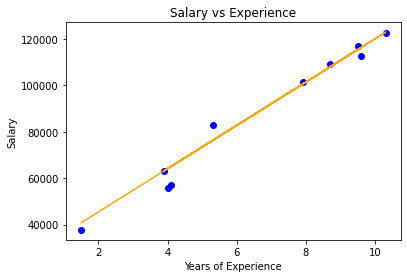# Implementation of Ridge Regression from Scratch using Python

• Last Updated : 18 Sep, 2020

Prerequisites:

1. Linear Regression

Introduction:
Ridge Regression ( or L2 Regularization ) is a variation of Linear Regression. In Linear Regression, it minimizes the Residual Sum of Squares ( or RSS or cost function ) to fit the training examples perfectly as possible. The cost function is also represented by J.

Cost Function for Linear Regression:Here, h(x(i)) represents the hypothetical function for prediction. y(i) represents the value of target variable for ith example.
m is the total number of training examples in the given dataset.

Linear regression treats all the features equally and finds unbiased weights to minimizes the cost function. This could arise the problem of overfitting ( or a model fails to perform well on new data ). Linear Regression also can’t deal with the collinear data ( collinearity refers to the event when the features are highly correlated ). In short, Linear Regression is a model with high variance. So, Ridge Regression comes for the rescue. In Ridge Regression, there is an addition of l2 penalty ( square of the magnitude of weights ) in the cost function of Linear Regression. This is done so that the model does not overfit the data. The Modified cost function for Ridge Regression is given below:Here, wj represents the weight for jth feature.
n is the number of features in the dataset.

Mathematical Intuition:
During gradient descent optimization of its cost function, added l2 penalty term leads to reduces the weights of the model to zero or close to zero. Due to the penalization of weights, our hypothesis gets simpler, more generalized, and less prone to overfitting. All weights are reduced by the same factor lambda. We can control the strength of regularization by hyperparameter lambda.

Different cases for tuning values of lambda.

1. If lambda is set to be 0, Ridge Regression equals Linear Regression
2. If lambda is set to be infinity, all weights are shrunk to zero.

So, we should set lambda somewhere in between 0 and infinity.

Implementation From Scratch:
Dataset used in this implementation can be downloaded from link
It has 2 columns — “YearsExperience” and “Salary” for 30 employees in a company. So in this, we will train a Ridge Regression model to learn the correlation between the number of years of experience of each employee and their respective salary. Once the model is trained, we will be able to predict the salary of an employee on the basis of his years of experience.

Code:

 # Importing libraries     import numpy as np  import pandas as pd  from sklearn.model_selection import train_test_split  import matplotlib.pyplot as plt     # Ridge Regression     class RidgeRegression() :             def __init__( self, learning_rate, iterations, l2_penality ) :                     self.learning_rate = learning_rate                  self.iterations = iterations                  self.l2_penality = l2_penality                 # Function for model training                  def fit( self, X, Y ) :                     # no_of_training_examples, no_of_features                  self.m, self.n = X.shape                     # weight initialization                  self.W = np.zeros( self.n )                     self.b = 0                 self.X = X                  self.Y = Y                     # gradient descent learning                             for i in range( self.iterations ) :                          self.update_weights()                      return self            # Helper function to update weights in gradient descent             def update_weights( self ) :                     Y_pred = self.predict( self.X )                     # calculate gradients                dW = ( - ( 2 * ( self.X.T ).dot( self.Y - Y_pred ) ) +                               ( 2 * self.l2_penality * self.W ) ) / self.m               db = - 2 * np.sum( self.Y - Y_pred ) / self.m                      # update weights              self.W = self.W - self.learning_rate * dW              self.b = self.b - self.learning_rate * db                  return self            # Hypothetical function  h( x )       def predict( self, X ) :              return X.dot( self.W ) + self.b         # Driver code     def main() :             # Importing dataset          df = pd.read_csv( "salary_data.csv" )      X = df.iloc[:, :-1].values      Y = df.iloc[:, 1].values             # Splitting dataset into train and test set      X_train, X_test, Y_train, Y_test = train_test_split( X, Y,                                                                                          test_size = 1 / 3, random_state = 0 )             # Model training          model = RidgeRegression( iterations = 1000,                                                           learning_rate = 0.01, l2_penality = 1 )      model.fit( X_train, Y_train )             # Prediction on test set      Y_pred = model.predict( X_test )          print( "Predicted values ", np.round( Y_pred[:3], 2 ) )           print( "Real values      ", Y_test[:3] )          print( "Trained W        ", round( model.W, 2 ) )          print( "Trained b        ", round( model.b, 2 ) )             # Visualization on test set           plt.scatter( X_test, Y_test, color = 'blue' )          plt.plot( X_test, Y_pred, color = 'orange' )          plt.title( 'Salary vs Experience' )          plt.xlabel( 'Years of Experience' )          plt.ylabel( 'Salary' )          plt.show()         if __name__ == "__main__" :       main()

Output:

Predicted values  [ 40831.44 122898.14  65078.42]
Real values       [ 37731 122391  57081]
Trained W         9325.76
Trained b         26842.8Visualization

Note: Ridge regression leads to dimensionality reduction which makes it a computationally efficient model.

My Personal Notes arrow_drop_up
Recommended Articles
Page :